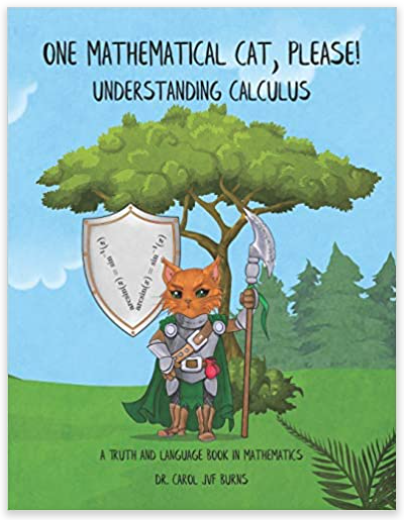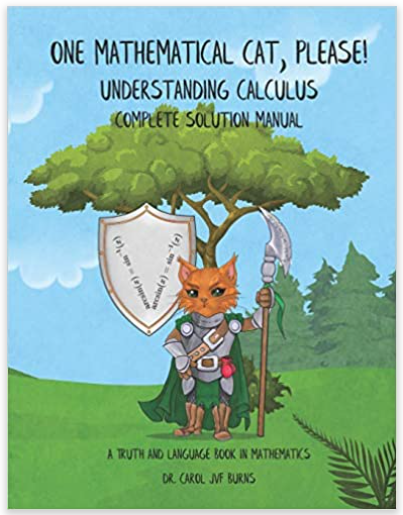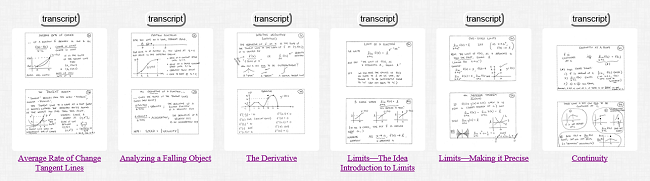# One Mathematical Cat, Please! Understanding Calculus

Want honest content, but a bit ‘slower and kinder’ than traditional Calculus books?
Like the way I explain things in Algebra I, Geometry, Algebra II, and Precalculus?
Then, this book is for you.

It's a complete Calculus course.
You can read it online (free, below) or buy a printed version.

I call it the ‘warrior cat’ book because of the print-version cover (look left)!
(You can read about these four cats in my Mathematical Cat Gallery:
numbers 23, 24, 25 and 26.)

Gone through my online curriculum through Precalculus?
Then, jump right into Chapter 3.

Haven't been using my lessons? No problem.
The first two chapters review essential material and introduce you to all the math language concepts you'll need.

It's a BIG file, and may take time to load into your browser.
So, you may prefer individual sections below.
However, it's certainly easier to search for stuff when it's all in one place.

## Print Version of the ‘Warrior Cat’ Book (and Complete Solution Manual) at Amazon

 The PRINT version of the Warrior Cat book is for those of us who like to highlight, write in margins, feel the paper between our fingers. Both the online and print versions include selected solutions: odd-numbered end-of-section exercises and Quick Quiz Problems. For ALL the solutions, I also offer a Complete Solution Manual. It includes completely-written-out solutions to: all the in-section exercises all the end-of-section exercises all the sample testsUnderstanding Calculus (the main text)Complete Solution Manual

## Calculus Index Cards

I also offer a set of Calculus Index Cards.
Hold the entire course in the palm of your hand!# READ THE ‘WARRIOR CAT’ BOOK:UNDERSTANDING CALCULUS

The materials in green give unlimited online practice and/or supplement the text.
PREFACE (iii–v)
ACKNOWLEDGEMENTS (vi)
TABLE OF SYMBOLS (viii–ix)
CHAPTER 2. FUNCTIONS
2.1 Functions and Function Notation (54–68)
2.2 Graphs of Functions (69–81)
2.3 Composite Functions (82–91)
2.4 One-to-One Functions and Inverse Functions (92–103)
SAMPLE TEST, Chapters 1 and 2 (104–107)
Prerequisites for Precalculus (with solutions): also needed for Calculus!
Average Rate of Change (great practice with function notation)
CHAPTER 3. LIMITS AND CONTINUITY
3.1 Limits—The Idea (108–119)

3.2 Limits—Making it Precise (120–132)
3.3 Properties of Limits (133–144)
3.4 Continuity (145–153)
3.5 Indeterminate Forms (154–159)
3.6 The Intermediate Value Theorem (160–170)
3.7 The Max-Min Theorem (171–178)
SAMPLE TEST, Chapter 3 (179–181)
CHAPTER 5. USING THE INFORMATION GIVEN BY THE DERIVATIVE
5.1 Increasing and Decreasing Functions (276–286)
5.2 Local Maxima and Minima—Critical Points (287–298)
5.3 The Second Derivative—Inflection Points (299–308)
5.4 Graphing Functions—Some Basic Techniques (309–319)
5.5 More Graphing Techniques (320–329)
5.6 Asymptotes—Checking Behavior at Infinity (330–338)
SAMPLE TEST, Chapter 5 (339–341)
CHAPTER 6. ANTIDIFFERENTIATION
6.1 Antiderivatives (342–353)
6.2 Some Basic Antidifferentiation Formulas (354–361)
6.3 Analyzing a Falling Object (optional) (362–375)
6.4 The Substitution Technique for Antidifferentiation (376–384)
6.5 More on Substitution (385–390)
6.6 Integration By Parts (391–397)
SAMPLE TEST, Chapter 6 (398–400)
CHAPTER 7. THE DEFINITE INTEGRAL
7.1 Using Antiderivatives to Find Area (401–407)
7.2 The Definite Integral (408–417)
7.3 The Definite Integral as the Limit of Riemann Sums (418–422)
7.4 The Substitution Technique applied to Definite Integrals (423–427)

7.5 The Area Between Two Curves (428–435)
7.6 Finding the Volume of a Solid of Revolution—Disks (436–443)
7.7 Finding the Volume of a Solid of Revolution—Shells (444–449)
SAMPLE TEST, Chapter 7 (450–452)
ABBREVIATED SOLUTIONS: Odd-Numbered End-of-Section Exercises and Quick Quiz Problems
Chapter 1 (453–457)
Chapter 2 (458–462)
Chapter 3 (463–469)
Chapter 4 (470–476)
Chapter 5 (477–479)
Chapter 6 (480–482)
Chapter 7 (483–486)
INDEX (487–497)